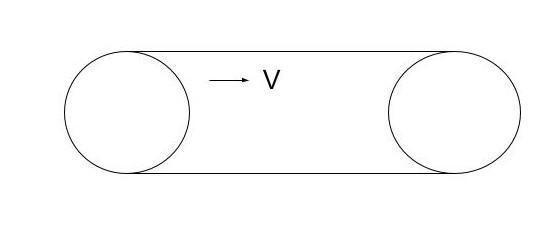Courses
Courses for Kids
Free study material
Offline Centres
MoreLast updated date: 06th Dec 2023
Total views: 280.2k
Views today: 3.80k

# The tractor is moving with velocity of 6km/hr. Mass of belt is 720gm .KE of belt isA. $4\,J$B. $1\,J$C. $2\,J$D. $6\,J$Verified
280.2k+ views
Hint: The kinetic energy of an object is the energy it has due to its motion in physics. It is the amount of work necessary to accelerate a body of a given mass from rest to a given velocity. If the body's speed varies, the kinetic energy gained during acceleration is retained. As the body decelerates from its current speed to a state of rest, it does the same amount of work.

Complete step-by-step solution:
In classical mechanics, the mass and speed of a point object (an object so small that its mass can be said to exist at one point) or a non-rotating rigid body determine its kinetic energy. The product of mass and speed squared equals half of the kinetic energy. In form of a formula:
$K.E = \dfrac{1}{2} \times m \times {v^2}$
Where $m$ is the mass and $v$ is the body's speed (or velocity). Mass is measured in kilogrammes, speed in metres per second, and kinetic energy is measured in joules in SI units.
Now let us come to the problem:
Given:
Mass of the belt is $m = 720\,gm$
Converting $m$ from $gm$ to $kg$$= \dfrac{{720}}{{1000}} = 0.72\,kg$
$\therefore m = 0.72\,kg$
Velocity of tractor is $v = 6km/hr.$
$v = 6 \times \dfrac{5}{{18}}m/s$
$v = \dfrac{5}{3}\,m/s$
$K.E = \dfrac{1}{2} \times m \times {v^2}$
$K.E = \dfrac{1}{2} \times 0.72 \times {\left( {\dfrac{5}{3}} \right)^2}$
$K.E = 1\,J$
So option $(2)$ is correct.

Note:Let us know something more about Kinetic energy. Windmills are an excellent example of kinetic energy applications. When wind (moving air) strikes the blades of a windmill, it causes them to rotate, resulting in the production of electricity.